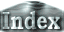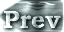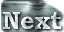# 4. Summary### b. Implications of the Present Study

The above conclusions suggest that the 1D radiative-convective equilibrium model is an extremely effective for describing the runaway greenhouse state. Therefore, it is considered that the results in Nakajima et al. (1992), as related to cases in which the dry air amount was changed, or where the absorption coefficient was varied, can be directly extended to the 3D system. Furthermore, the conclusions reached in Kasting (1988) and Abe and Matsui (1988) on the evolution of primitive atmosphere can be expected to be generally consistent with the results of similar studies in 3D. However, as they pointed out in their studies, the upper limit of outgoing infrared radiation at the top of the atmosphere would most likely be higher than the values they calculated if relative humidity were to be considered. Therefore, a slight increase in the value of the accumulation energy flux for forming the primitive ocean calculated in Abe and Matsui (1988) can be expected.

Nakajima et al. (1992) found solutions which give same values of outgoing radiation flux at different surface temperatures, which implies that multiple equilibrium solutions exist depending on the incident solar radiation flux. However, the stability of such equilibrium solutions has not been fully studied. The results of the present study favor the equilibrium solution for the lower surface temperature in Nakajima et al. (1992) as the stable solution. However, the stability of the equilibrium solution for the higher surface temperature has not been confirmed, even though it is predicted to be unstable. The time evolution problem must be solved in order to reach a conclusion, because various time scales, such as the radiation relaxation time and the time scale of water vapor distribution variation, must be known for it to be calculated. One method for resolving this problem is to use the results of S1600 in the experiment as the initial values, and to give a lower value for incident solar radiation flux to confirm whether an equilibrium state is reached. These will be themes for future studies.

 4.b. Implications of the Present Study